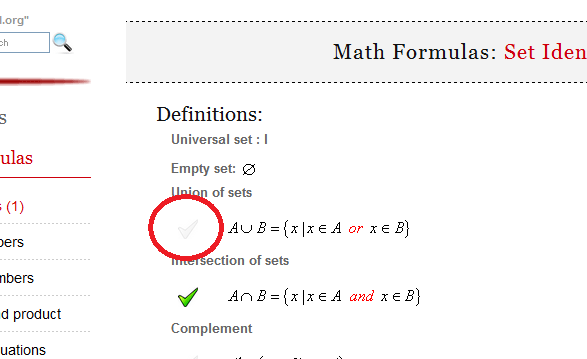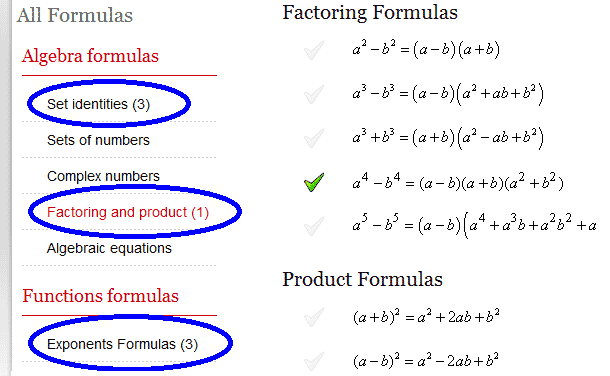Math Calculators, Lessons and Formulas

It is time to solve your math problem

mathportal.org

You included 0 formulas in your custom cheat sheet.

Cheat Sheet options

## How to generate cheat sheet ?

To create cheat sheet first you need to select formulas which you want to include in it. To select formula click at picture next to formula.You can choose formulas from different pages.After you have selected all the formulas which you would like to include in cheat sheet, click the "Generate PDF" button.# Math Formulas: Complex numbers

### Definitions:

A complex number is written as $a + b\,i$ where $a$ and $b$ are real numbers an $i$, called the imaginary unit, has the property that $i^2 = -1$.

The complex numbers $z = a + b\,i$ and $\overline{z} = a - b\,i$ are called complex conjugate of each other.

### Formulas:

Equality of complex numbers

 $$a + b\,i = c + d\,i \Longleftrightarrow a = c ~~and~~ b = d$$

 $$(a + b\,i) + (c + d\,i) = (a + c) + (b + d)\,i$$

Subtraction of complex numbers

 $$(a + b\,i) - (c + d\,i) = (a - c) + (b - d)\,i$$

Multiplication of complex numbers

 $$(a + b\,i)\cdot(c + d\,i) = (ac - bd) + (ad + bc)\,i$$

Division of complex numbers

 $$\frac{a + b\,i}{c + d\,i} = \frac{a + b\,i}{c + d\,i}\cdot\frac{c - d\,i}{c - d\,i} = \frac{ac + bd}{c^2 + d^2} + \frac{bc - ad}{c^2 + d^2} \, i$$

Polar form of complex numbers

 $$a + b\,i = r\cdot(\cos\theta + i\,\sin\theta)$$

Multiplication and division of complex numbers in polar form

 $$\left[r_1\left(\cos\theta_1 + i \cdot \sin\theta_1\right)\right] \cdot \left[r_2\left(\cos\theta_2 + i \cdot \sin\theta_2\right)\right] = r_1 \cdot r_2 \left[ \cos\left(\theta_1+\theta_2\right) + i \cdot \sin\left(\theta_1+\theta_2\right) \right]$$
 $$\frac{r_1\left(\cos\theta_1 + i\,\sin\theta_1\right)}{r_2\left(\cos\theta_2 + i\,\sin\theta_2\right)}= \frac{r_1}{r_2} \left[\cos\left(\theta_1-\theta_2\right) + i \cdot \sin\left(\theta_1-\theta_2\right)\right]$$

De Moivre's theorem

 $$\left[r \left( \cos\theta + i\,\sin\theta \right) \right]^n = r^n \left( \cos ( n\theta) + i\,\sin (n\theta) \right)$$

Roots of complex numbers

 $$\left[r \left( \cos\theta + i\,\sin\theta \right) \right]^{1/n} = r^{1/n} \left( \cos \frac{\theta + 2k\pi}{n} + i\,\sin \frac{\theta + 2k\pi}{n} \right) ~~ k=0,1,\dots, n-1$$